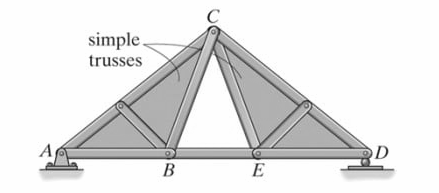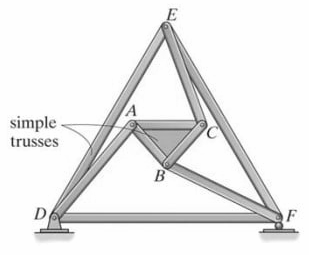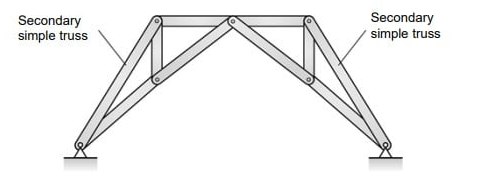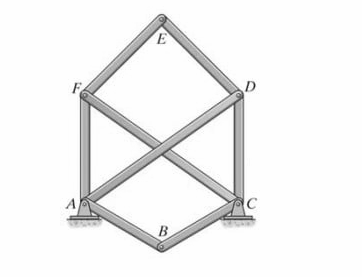Select Page

# Trusses

Trusses

Contents

Define and differentiate between the terms Trusses and Frames 1

Classify Truss 2

Recall the assumptions used in the analysis of trusses 6

Recall the Sign convention used for the analysis of trusses 7

Describe the general procedure for the analysis of truss members by using Method of Joints 8

Describe the general procedure for the analysis of truss members by using the Method of Sections 9

Describe the general procedure for the analysis of truss members by using the Tension Coefficient Method 10

# Define and differentiate between the terms Trusses and Frames

The terms “Trusses” and “Frames” are both structural systems used in the design of buildings and other structures to provide support and stability. However, there are some important differences between the two that are worth noting.

Trusses:

A truss is a structural element made up of interconnected triangles of structural members, usually made of steel or timber. The members are connected by joints at the endpoints, and these joints are usually pinned or riveted. The design of a truss allows it to transfer loads efficiently along its length, making it well-suited for spanning long distances without intermediate support. Trusses are commonly used in bridges, roofs, and towers, and they are prized for their ability to provide a strong, lightweight structure.

Frames:

Frames, on the other hand, are structures composed of a series of interconnected members that span between supports. Unlike trusses, which rely on triangles for their strength, frames can have a variety of shapes and can be made up of members with different cross-sectional shapes and sizes. Frames are typically made of steel, concrete, or timber, and they can be designed to resist both vertical and horizontal loads. Unlike trusses, frames are less efficient at spanning long distances and typically require intermediate supports. However, frames offer more design flexibility and can be used in a wider range of structures, including buildings and machinery.

The key difference between trusses and frames is the way that they transfer loads. Trusses rely on a series of interconnected triangles to transfer loads along their length, while frames use a variety of interconnected members to span between supports. As a result, trusses are more efficient at spanning long distances without intermediate support, while frames offer more design flexibility and can be used in a wider range of structures. Another difference is that trusses are usually made of steel or timber, while frames can be made of a variety of materials, including steel, concrete, and timber.

# Classify Truss

Co-planar trusses are classified into three types which are

• Simple Truss
• Compound Truss
• Complex Truss

Simple Truss

• The simplest framework that is rigid or stable is a triangle.
• Therefore, a simple truss is constructed starting with a basic triangular element and connecting two members to form additional elements.
• As each additional element of two members is placed on a truss, the number of joints is increased by one.

Compound Truss

• This truss is formed by connecting two or more simple trusses together.
• This type of truss is often used for large spans.

There are three ways in which simple trusses may be connected to form a compound truss:

1. Trusses may be connected by a common joint and bar.\2. Trusses may be joined by three bars.3. Trusses may be joined where bars of a large simple truss, called the main truss, have been substituted by simple trusses, called secondary trusses4. Complex Truss

This is a truss that cannot be classified as being either simple or compound.# Recall the assumptions used in the analysis of trusses

The analysis of trusses involves using mathematical and engineering principles to predict the behavior of the structure under various loads and conditions. To simplify this analysis, certain assumptions are made about the behavior of the truss, including:

1. Pin-Jointed Members: It is assumed that the joints in a truss are pinned, meaning that they only allow rotation and do not allow any translation. This simplifies the analysis of the truss and allows the forces in the members to be determined using the principles of statics.
2. Two-Dimensional Structure: Trusses are assumed to be two-dimensional structures, meaning that the members only experience forces in two directions (vertical and horizontal). This simplifies the analysis and makes it easier to predict the behavior of the structure under load.
3. Linear Elastic Material: The material used to construct the truss is assumed to be linear and elastic, meaning that it deforms proportionally to the applied load and returns to its original shape when the load is removed. This simplifies the analysis and allows engineers to use established equations and formulas to predict the behavior of the truss under load.
4. No Member Axial Deformation: It is assumed that the members of the truss will not deform axially, meaning that they will not change length under load. This simplifies the analysis and ensures that the truss will maintain its overall shape and stability under load.
5. No Member Slip or Yield: It is also assumed that the members will not slip or yield, meaning that they will not move or deform at the joints under load. This simplifies the analysis and ensures that the truss will maintain its stability and integrity under load.

These assumptions are used in the analysis of trusses to simplify the calculations and make it possible to predict the behavior of the structure under load. Understanding these assumptions and their limitations is important for engineers and designers in the design and analysis of trusses.

# Recall the Sign convention used for the analysis of trusses

The sign convention used in the analysis of trusses is a set of rules that defines the direction of forces and the positive and negative directions for load, force, and displacement. The sign convention used for truss analysis is based on the convention used in engineering mechanics and is designed to simplify the analysis and ensure consistent results. The following are the most common sign conventions used in the analysis of trusses:

1. Load direction: Loads applied to the truss are assumed to act in the downward direction and are assigned a negative sign. This makes it easier to identify the direction of the loads and to determine the net force in the structure.
2. Member force direction: Forces in the members of the truss are assumed to act in the direction of the member and are assigned a positive sign if they are in tension and a negative sign if they are in compression. This makes it easy to identify the direction of the forces and to determine the net force in the structure.
3. Member orientation: Members are assumed to be oriented vertically, with the bottom of the member assigned a negative sign and the top of the member assigned a positive sign. This makes it easier to identify the orientation of the members and to determine the net force in the structure.
4. Displacement direction: Displacement is assumed to act in the upward direction and is assigned a positive sign. This makes it easier to identify the direction of displacement and to determine the net force in the structure.

These sign conventions are used to simplify the analysis of trusses and to ensure consistent results. Understanding the sign convention used for the analysis of trusses is important for engineers and designers in the design and analysis of truss structures.

# Describe the general procedure for the analysis of truss members by using Method of Joints

The Method of Joints is a commonly used procedure for the analysis of truss members. It is a systematic approach that allows engineers to determine the forces in each member of a truss and to predict the behavior of the structure under load. The general procedure for the analysis of truss members using the Method of Joints is as follows:

1. Determine the loads acting on the truss: The first step in the analysis of truss members is to determine the loads acting on the truss. This includes both point loads and uniform loads. Point loads are loads that are applied at a single point, while uniform loads are loads that are spread out over a longer span.
2. Identify the joints: The next step is to identify the joints in the truss. Joints are the points where the members of the truss intersect and are typically where the loads are applied.
3. Draw a free body diagram for each joint: For each joint, a free body diagram is drawn to represent the forces acting on that joint. The free body diagram should include all of the forces acting on the joint, including the loads and the forces in the members.
4. Determine the unknown forces in the members: Using the free body diagram and the principles of statics, the unknown forces in the members can be determined. This involves solving for the forces in the members using equations and using the sign convention to determine the direction of the forces.
5. Repeat for each joint: The above steps are repeated for each joint in the truss, allowing the forces in all of the members to be determined.
6. Check for equilibrium: Once the forces in all of the members have been determined, the equilibrium of the truss can be checked by verifying that the sum of the forces and moments acting on the truss is equal to zero.
7. Determine the deformations and stresses in the members: The final step in the analysis of truss members is to determine the deformations and stresses in the members. This involves using the forces determined in the previous steps and the material properties of the members to predict the behavior of the structure under load.

The Method of Joints is a straightforward and systematic approach to the analysis of truss members. By following this procedure, engineers and designers can determine the forces in each member of a truss and predict the behavior of the structure under load.

# Describe the general procedure for the analysis of truss members by using the Method of Sections

The Method of Sections is a commonly used procedure for the analysis of truss members. It is a systematic approach that allows engineers to determine the forces in each member of a truss and to predict the behavior of the structure under load. The general procedure for the analysis of truss members using the Method of Sections is as follows:

1. Determine the loads acting on the truss: The first step in the analysis of truss members is to determine the loads acting on the truss. This includes both point loads and uniform loads. Point loads are loads that are applied at a single point, while uniform loads are loads that are spread out over a longer span.
2. Identify the section: The next step is to identify a section in the truss that contains the members whose forces are to be determined. This section should include both internal and external members, as well as all of the joints on either side of the section.
3. Draw a free body diagram of the section: A free body diagram is then drawn to represent the forces acting on the section. The free body diagram should include all of the forces acting on the section, including the loads and the forces in the members.
4. Cut the members: The members in the section are then cut and the internal forces in these members are assumed to be zero. This allows the internal forces in these members to be determined from the forces acting on the section.
5. Determine the unknown forces in the members: Using the free body diagram and the principles of statics, the unknown forces in the members can be determined. This involves solving for the forces in the members using equations and using the sign convention to determine the direction of the forces.
6. Repeat for each section: The above steps are repeated for each section in the truss, allowing the forces in all of the members to be determined.
7. Check for equilibrium: Once the forces in all of the members have been determined, the equilibrium of the truss can be checked by verifying that the sum of the forces and moments acting on the truss is equal to zero.
8. Determine the deformations and stresses in the members: The final step in the analysis of truss members is to determine the deformations and stresses in the members. This involves using the forces determined in the previous steps and the material properties of the members to predict the behavior of the structure under load.

The Method of Sections is a straightforward and systematic approach to the analysis of truss members. By following this procedure, engineers and designers can determine the forces in each member of a truss and predict the behavior of the structure under load. This method is particularly useful when the truss contains a large number of members or when it is not feasible to use the Method of Joints.

# Describe the general procedure for the analysis of truss members by using the Tension Coefficient Method

The Tension Coefficient Method, also known as the Slope-Deflection Method, is a procedure used for the analysis of truss members that involves calculating the deflections and rotations at each joint due to loads on the truss. The general procedure for the analysis of truss members using the Tension Coefficient Method is as follows:

1. Determine the loads acting on the truss: The first step in the analysis of truss members is to determine the loads acting on the truss. This includes both point loads and uniform loads. Point loads are loads that are applied at a single point, while uniform loads are loads that are spread out over a longer span.
2. Determine the stiffness matrix for the truss: The stiffness matrix for the truss is then calculated by determining the coefficients that relate the forces in each member to the deflections and rotations at the joints.
3. Solve for the nodal deflections and rotations: Using the stiffness matrix, the nodal deflections and rotations can be calculated by solving a set of linear equations. This step involves determining the relationship between the loads on the truss and the deflections and rotations at each joint.
4. Solve for the member forces: Once the nodal deflections and rotations have been determined, the member forces can be calculated. This involves determining the forces in each member based on the deflections and rotations at the joints.
5. Check for equilibrium: The equilibrium of the truss can be checked by verifying that the sum of the forces and moments acting on the truss is equal to zero.
6. Determine the deformations and stresses in the members: The final step in the analysis of truss members is to determine the deformations and stresses in the members. This involves using the forces determined in the previous steps and the material properties of the members to predict the behavior of the structure under load.

The Tension Coefficient Method is a powerful tool for the analysis of truss members, particularly when the truss contains a large number of members or when it is not feasible to use the Method of Joints or the Method of Sections. This method allows engineers to calculate the deflections and rotations at each joint, which provides important information about the behavior of the truss under load. Additionally, the Tension Coefficient Method can be used to analyze the effects of member redundancy and the stability of the truss.